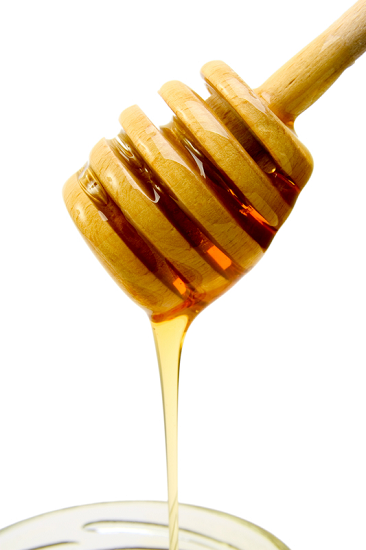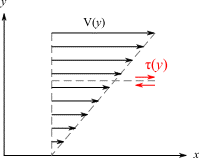# Difference Between Kinematic and Dynamic Viscosity

## Introduction

If you have ever tried to take out honey by keeping the bottle upside down, it feels thick. It's due to the high viscosity of honey. And while pouring ketchup from the bottle, it takes more time to flow and needs an extra instantaneous force (by squeezing or squirting). This is because ketchup is also very thick. But viscosity in play is not the same in both cases. In the first case, there is Kinematic Viscosity When only gravitational force is in action, and in the second case, when you apply an external force by squeezing, dynamic viscosity is in play. Now let's see what is viscosity in detail and what is the difference between kinematic viscosity and dynamic viscosity. Their units, representation, and the relationship between them.## What is Viscosity?

In fluids (liquid or gas), resistance (or friction) to flow is known as viscosity. It arises due to the interactions between the entities of fluids. A given temperature and pressure are characteristic of a given fluid. Viscosity is of two types kinematic viscosity and dynamic viscosity. Viscosity differs with temperature for a given fluid. In liquids, generally, it increases with temperature and in gases, decreases with temperature. Mathematically, the viscosity is estimated by the given formula

$\mathrm{\eta=\frac{shear\:stress}{velocity \:gradient}}$

The shear stress is the ratio of force to the area. And velocity gradient $\mathrm{\frac{dv}{dx}}$ is the rate at which the velocity is changed. Hence, the formula is given by

$\mathrm{\eta=\frac{F/A}{dv/dx}}$

$\mathrm{\eta=\frac{F.dv}{A.dx}}$

Where $\mathrm{\eta=coefficient\:of \:viscosity.}$

F is force and A is area.

$\mathrm{\frac{dv}{dx}}$ is velocity gradient.

## What is Kinematic Viscosity?

When a fluid is flowing under gravitational force only, the resistance to flow against the gravitational force is known as kinematic viscosity. It depends on mass density which is the mass of the fluid over the volume of the fluid. It's measured as the dynamic viscosity over the mass density. It's related to the overall flow of fluid under gravity only.

## Units and Dimensions of Kinematic Viscosity

Units of kinematic viscosity are $\mathrm{m^2 sec^{-1}}$

The CGS unit is stoke is still widely used:

$\mathrm{1\:stoke=10^{-4} m^2 sec^{-1}}$

$\mathrm{1\:stoke=10^{2} \:centistoke}$

The dimensions are $\mathrm{[M^0 L^2 T^{-1}]}$

## Representation and Formula for Kinematic Viscosity

Kinematic Viscosity is represented by the Greek letter ν pronounced as "nu". And the formula for the kinematic viscosity of a liquid is given by

$\mathrm{v=\frac{\mu}{\rho}}$

Where ν=kinematic viscosity

$\mathrm{\mu}$ dynamic viscosity

$\mathrm{\rho}$ density of liquid

From the above expression, we observe that kinematic viscosity is the ratio of the dynamic viscosity to the density of the liquid.

## What is Dynamic Viscosity?

It is the ratio of shear stress (force per unit area) over the velocity gradient. In a steady flow of a fluid past a solid surface, the rate at which the velocity changes with distance within a limiting distance from the surface is called the velocity gradient. It's related to internal resistance in a fluid among the neighborhood particles under an external force other than gravity.Fig:2 Dynamic viscosity

## Units and Dimensions of Dynamic Viscosity

The SI physical unit of dynamic viscosity is the Pascal-second, and the CGS unit is the poise (P), named after Jean Poiseuille.

1 poise = 0.1 Pascal second

1 poise = 100 centipoise

The dimensions formula of dynamic viscosity is $\mathrm{[M^1 L^{-1} T^{-1}]}$

### Representation and Formula for Dynamic Viscosity

We know that dynamic viscosity is a force that helps the liquid molecules to overcome internal friction. So it is equivalent to the ratio of shearing stress to the shear rate change.

$\mathrm{\mu=\frac{T}{Y}}$

Where $\mathrm{\mu}$ is the dynamic viscosity

T is shearing stress

Y is a shearing rate change

## Relation of Kinetic and Dynamic Viscosity with Density

The kinetic viscosity is given by

$\mathrm{v=\frac{\mu}{\rho}}$

we observe that kinetic viscosity is inversely related to density which means if the density is increased the kinetic viscosity decreases and vice versa.

The dynamic viscosity is given by

$\mathrm{\mu=\frac{T}{Y}}$

From the above equation, we conclude that kinetic viscosity has a direct relation with dynamic viscosity and an inverse relation with density. This means dynamic viscosity is also directly related to density.

## Application of Viscosity

• The knowledge of viscosity helps us to understand which lubricant or oil is suitable for machines and engines.

• The coefficient of viscosity is used to calculate the molecular weight of organic liquids.

• It also finds the applications in the pharmaceutical industry in the manufacturing of medicines like cough syrup.

• Viscosity also plays an important role in the manufacturing of paints and varnishes. The coefficient of viscosity is also maintained in them so it can be easy to apply to the walls.

• It is a characteristic when choosing the cooking oil, that directly affects our health.

• Viscosity also finds applications in manufacturing printing ink.

## Conclusion

Kinematic Viscosity comes into play when we talk about the overall flow of fluid under gravity only. Dynamic Viscosity comes into play when we talk about the internal resistance in fluid among its neighbouring particles under an external force other than the gravitational force. In this tutorial, we learned that viscosity is of two types i.e. kinematic and dynamic viscosity. And how these are related to densities and their application in different industries as well as in our daily life.

## FAQs

Q1. What is absolute viscosity?

Ans: The ratio of shear stress (force per unit area) over the velocity gradient is known as dynamic viscosity. And it's also known as Absolute viscosity.

Q2. How does kinematic viscosity depend on temperature?

Ans: Viscosity depends on temperature and a change in temperature changes the volume of fluid and so the density of the fluid. And thus change in viscosity of the given fluid.

Q3. What is the significance of kinematic viscosity?

Ans: Kinematic viscosity signifies the property of a fluid to flow through a cross-section of a given area in a given time at constant temperature under gravitational force only.

Q4. What is the significance of dynamic viscosity?

Ans: Dynamic viscosity signifies the property of a fluid to flow through a cross-section of a given area in a given time at constant temperature under an external force other than the gravitational force.

Q5. What are Newtonian fluid and non-Newtonian fluid?

Ans: For an ideal fluid, the viscosity remains constant at constant temperature ie., It does not change under shear stress ( or pressure ) at constant temperatures, like water. But in non-newtonian fluid, like ketchup, its viscosity changes dramatically under shear stress for a short period even when the temperature remains the same.

Updated on: 10-Jan-2023

267 Views